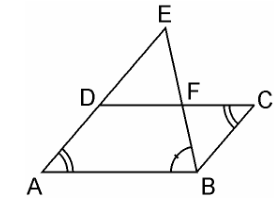Guru

# E is a point on the side AD produced of a parallelogram ABCD and BE intersects CD at F. Show that ΔABE ~ ΔCFB. Q.8

• 0

The best way to solve the question of class 10th math of triangles chapter of exercise 6.3, how i solve this question, because it is very tough E is a point on the side AD produced of a parallelogram ABCD and BE intersects CD at F. Show that ΔABE ~ ΔCFB.

Share

1. Given, E is a point on the side AD produced of a parallelogram ABCD and BE intersects CD at F. Consider the figure below,In ΔABE and ΔCFB,

∠A = ∠C (Opposite angles of a parallelogram)

∠AEB = ∠CBF (Alternate interior angles as AE || BC)

∴ ΔABE ~ ΔCFB (AA similarity criterion)

• 0